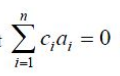GATE | GATE-CS-2017 (Set 1) | Question 21

• Last Updated : 28 Jun, 2021

Let c1, cn be scalars not all zero. Such that the following expression holds:where ai is column vectors in Rn.
Consider the set of linear equations.
Ax = B.
where A = [a1…….an] and

(A) a unique solution has x = jn where j denotes n dimensional vector for all 1.
(B) no solution
(C) infinitely many solution
(D) finitely many solution

Explanation: The vectors a1, a2, …, an are linearly dependent.
For the system AX = B,
Rank of coefficient matrix A = Rank of augmented matrix (A / B)
= k (k< n)
Hence, the system has infinitely many solutions.

This solution is contributed by Sumouli Chaudhary.

Quiz of this Question

My Personal Notes arrow_drop_up